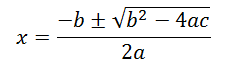# C Program to Find the Roots of Quadratic Equation

A quadratic equation is an equation of the form ax2 + bx + c = 0 where a, b and c are constants.

And the formula to calculate the roots of the quadratic equation is:C program to find the roots of a quadratic equation is shown below.

```#include<stdio.h>
#include<math.h>

int main()
{
float a, b, c, x, d, r1, r2;
printf (“Enter the values of a, b and c n”);
scanf (“%f %f %f”, &a, &b, &c);
x = (b*b) – 4 * a * c;
d = sqrt (fabs(x));

if (x==0)
{
r1 = - b / 2 * a;
r2 = - b / 2 * a;
printf (“n The roots are real and equal and the roots are %f  %f”,r1,r2);
}

else if (x > 0)
{
r1 = (-b + d) / 2 * a;
r2= (-b – d) / 2 * a;
printf (“The roots are real and unequal and the roots are %f %f “, r1, r2);
}

else
{
r1 = (-b + d) / 2 * a;
r2= (-b – d) / 2 * a;
printf (“The roots are imaginary and unequal and the roots are %f %fi “, r1, r2);
}

return 0;

}
```

The values a, b and c are entered by the user. Then the value of x (i.e. the value of b2-4ac) is calculated. The keyword sqrt() calculates the square root. The value ofis calculated by the statement sqrt (fabs(x)) where fabs means absolute value of float. This value is assigned to variable d. Now, if the value of x is 0 then the process associated with the if condition is executed. If the value of x is greater than 0 then the process associated with the else if condition is executed, otherwise the value associated with the else condition is executed.

This program can also be executed by using the switch statement.

```#include<stdio.h>
#include<math.h>

int main()
{
float a,b,c,x,d,r1,r2;
int z;
printf ("Enter the values of a, b and c n");
scanf ("%f %f %f", &a, &b, &c);
x = (b*b) - 4 * a * c;
d = sqrt (fabs(x));

if (d==0)
z=1;
else if (d>0)
z=2;
else
z = 3;

switch (z)
{
case 1 : r1 = - b / 2 * a;
r2 = - b / 2 * a;

printf ("n The roots are real and equal and the roots are %f  %f",r1,r2);
break;

case 2 : r1 = (-b + d) / 2 * a;
r2= (-b - d) / 2 * a;

printf ("The roots are real and unequal and the roots are %f %f ", r1, r2);

break;

case 3 : r1 = (-b + d) / 2 * a;
r2= (-b - d) / 2 * a;

printf ("The roots are imaginary and unequal and the roots are %f %fi ", r1, r2);

break;
}

return 0;

}
```

Here if the value of d is zero, then z=1 and if d is greater than zero, then z = 2 otherwise the value of z equals 3. Now the value of z is checked using the switch statement. If the value of z is 1, then the statements associated with case 1 label is executed. If the value of z is 2 then the statements associated with the case 2 label is executed. And finally, if the value of z is 3 then the statements associated with the case 3 label is executed.

Output

```Enter the values of a, b and c
1
6
-7

The roots are real and unequal and the roots are 1.00 and -7.00```